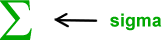OK, so we know what a sequence is -- it's a list of numbers (or other things) that changes according to some pattern.

So, what's a series?  Easy!

A SERIES is the sum of a sequence.

Here's a sequence:Here's the corresponding series:We have a special notation for series.

First, let's get the formula for the nth term of the above sequence...
I think it'sDo you agree?  Does it work?

Find the first five terms to check.

So, how are we going to let people know that we want to add up all the terms of this sequence and make it a series?

We'll use this Greek letter:It's an "S" in the Greek alphabet.
Think of it as an "S" for "sum!"